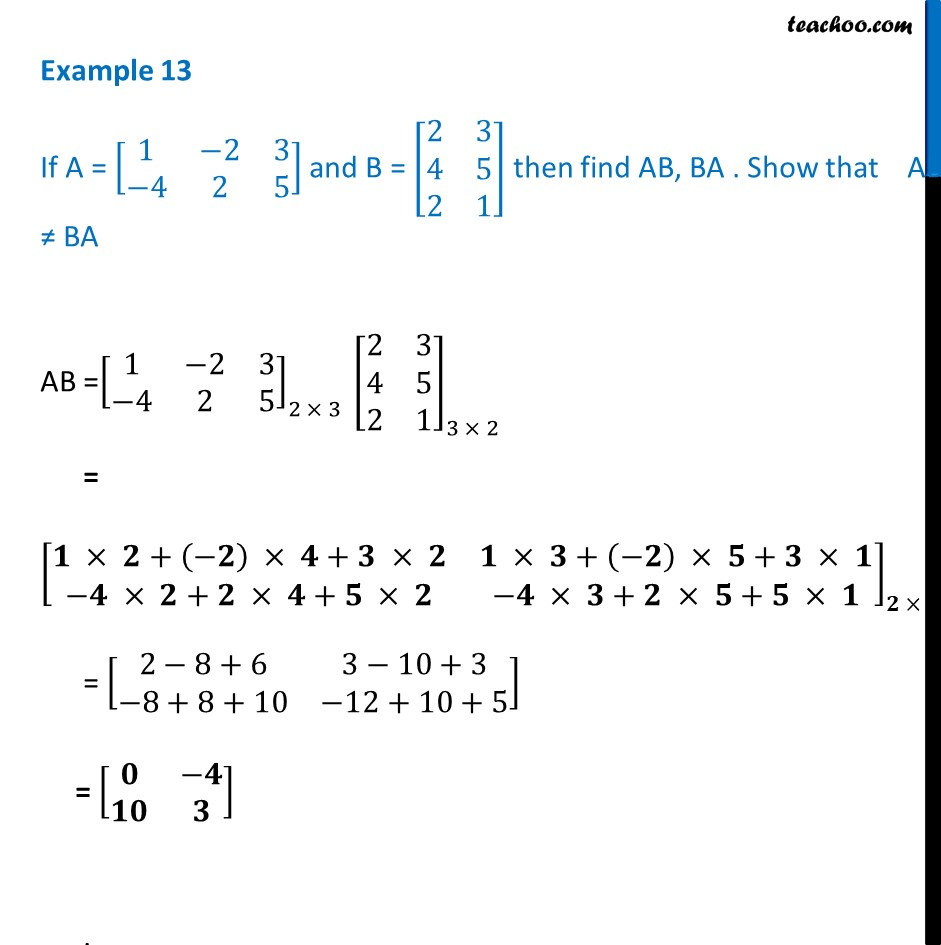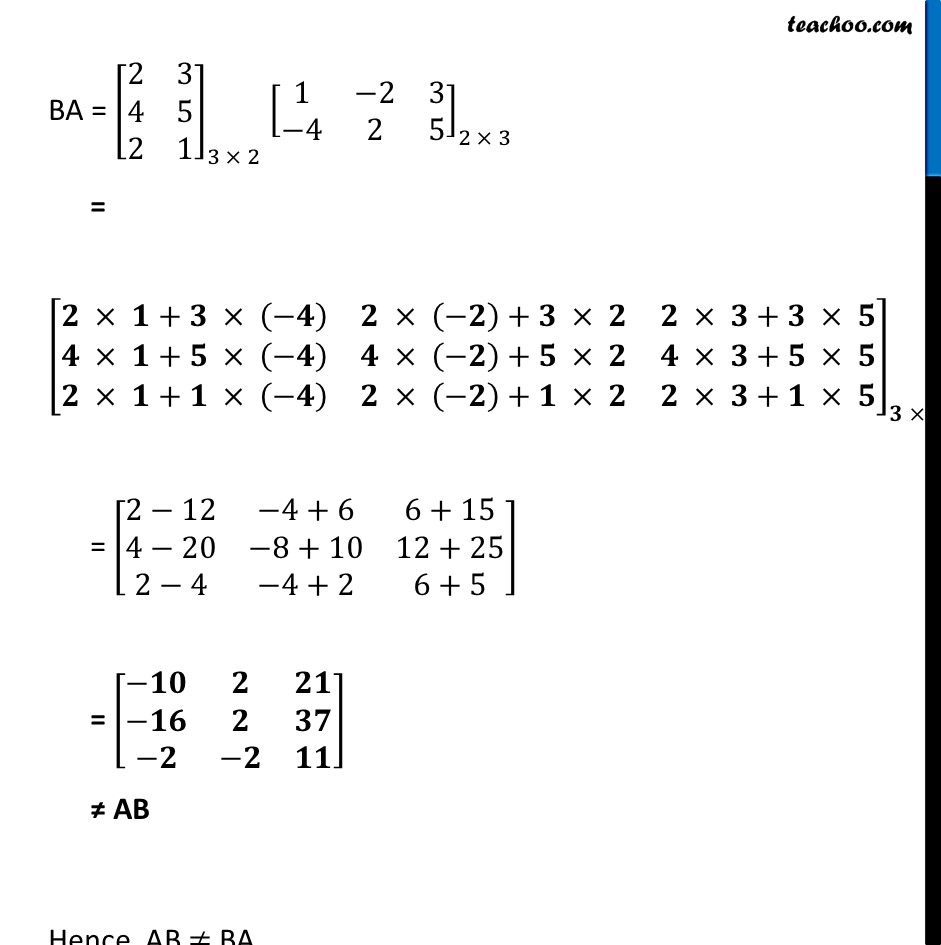Examples

Chapter 3 Class 12 Matrices
Serial order wiseLearn in your speed, with individual attention - Teachoo Maths 1-on-1 Class

### Transcript

Example 13 If A = [■8(1&−2&3@−4&2&5)] and B = [■8(2&3@4&5@2&1)] then find AB, BA . Show that AB ≠ BA AB =[■8(1&−2&3@−4&2&5)]_(2 × 3 ) [■8(2&3@4&5@2&1)]_(3 × 2) = [■8(𝟏 × 𝟐+(−𝟐) × 𝟒+𝟑 × 𝟐&𝟏 × 𝟑+(−𝟐) × 𝟓+𝟑 × 𝟏@−𝟒 × 𝟐+𝟐 × 𝟒+𝟓 × 𝟐&−𝟒 × 𝟑+𝟐 × 𝟓+𝟓 × 𝟏)]_(𝟐 × 𝟐 ) = [■8(2−8+6&3−10+3@−8+8+10&−12+10+5)] = [■8(𝟎&−𝟒@𝟏𝟎&𝟑)] . BA = [■8(2&3@4&5@2&1)]_(3 × 2) [■8(1&−2&3@−4&2&5)]_(2 × 3) = [■8(𝟐 × 𝟏+𝟑 × (−𝟒)&𝟐 × (−𝟐)+𝟑 × 𝟐&𝟐 × 𝟑+𝟑 × 𝟓@𝟒 × 𝟏+𝟓 × (−𝟒)&𝟒 × (−𝟐)+𝟓 × 𝟐&𝟒 × 𝟑+𝟓 × 𝟓@𝟐 × 𝟏+𝟏 × (−𝟒)&𝟐 × (−𝟐)+𝟏 × 𝟐&𝟐 × 𝟑+𝟏 × 𝟓)]_(𝟑 × 𝟑 ) = [■8(2−12&−4+6&6+15@4−20&−8+10&12+25@2−4&−4+2&6+5)] = [■8(−𝟏𝟎&𝟐&𝟐𝟏@−𝟏𝟔&𝟐&𝟑𝟕@−𝟐&−𝟐&𝟏𝟏)] ≠ AB Hence AB ≠ BA